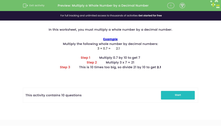# Multiply a Whole Number by a Decimal Number

In this worksheet, students will multiply a whole number by a simple decimal number.Key stage:  KS 3

Curriculum topic:   Number

Curriculum subtopic:   Use Four Operations for All Numbers

Difficulty level:#### Worksheet Overview

In this activity, we will multiply a whole number by a decimal number.

Example

Do the following multiplication:

3 x 0.7 = 2.1

The easiest way to multiply decimals, is to remove the decimal point, do the multiplication and then add the decimal point back into our answer.

3 x 0.7

Remove the decimal point: 3 x 7

Do the multiplication: 3 x 7 = 21

Replace the decimal point: 2.1

The only tricky part is knowing where to put the decimal point in your answer.

The key to this is to count how many decimal places there are in the original calculation and make sure there's the same number in your answer!

In 3 x 0.7, there is one decimal place, so the answer must also have one decimal place.

So 21 becomes 2.1

Easy, isn't it?### What is EdPlace?

We're your National Curriculum aligned online education content provider helping each child succeed in English, maths and science from year 1 to GCSE. With an EdPlace account you’ll be able to track and measure progress, helping each child achieve their best. We build confidence and attainment by personalising each child’s learning at a level that suits them.

Get started